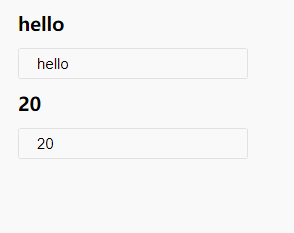# react-hooks-input-bind

React hook for creating input values. Implement two-way binding of data.

## Usage no npm install needed!

``````<script type="module">
import reactHooksInputBind from 'https://cdn.skypack.dev/react-hooks-input-bind';
</script>``````

## react-hooks-input-bind

React hook for creating input values. Implement two-way binding of data.

Note: This is using the new React Hooks API Proposal which is subject to change until React 16.7 final. You'll need to install react, react-dom, etc at ^16.7.0-alpha.0

### Install

``````npm install react-hooks-input-bind
npm install react@^16.7.0-alpha.0 react-dom@^16.7.0-alpha.0
``````

### Usage

``````import React from 'react';
import useBind from 'react-hooks-input-bind';

export default () => {
const [state, bind] = useBind({
name: 'hello',
age: '20',
});

return (
<div>
<h3>{state.name}</h3>
<input {...bind.name} />

<h3>{state.age}</h3>
<input {...bind.age} />
</div>
)
}
``````The initial value can also be a string, an array, a numeric value, and so on.

``````const [state, bind] = useBind('hello');
<h3>{state}</h3>
<input {...bind} />

const [state, bind] = useBind(20);
<h3>{state}</h3>
<input {...bind} />

const [state, bind] = useBind(['hello', 20]);
<h3>{state}</h3>
<h3>{state}</h3>

<input {...bind} />
<input {...bind} />
``````